Meta-RL is meta-learning on reinforcement learning tasks. After trained over a distribution of tasks, the agent is able to solve a new task by developing a new RL algorithm with its internal activity dynamics. This post starts with the origin of meta-RL and then dives into three key components of meta-RL.

In my earlier post on meta-learning, the problem is mainly defined in the context of few-shot classification. Here I would like to explore more into cases when we try to “meta-learn” Reinforcement Learning (RL) tasks by developing an agent that can solve unseen tasks fast and efficiently.

To recap, a good meta-learning model is expected to generalize to new tasks or new environments that have never been encountered during training. The adaptation process, essentially a mini learning session, happens at test with limited exposure to the new configurations. Even without any explicit fine-tuning (no gradient backpropagation on trainable variables), the meta-learning model autonomously adjusts internal hidden states to learn.

Training RL algorithms can be notoriously difficult sometimes. If the meta-learning agent could become so smart that the distribution of solvable unseen tasks grows extremely broad, we are on track towards general purpose methods — essentially building a “brain” which would solve all kinds of RL problems without much human interference or manual feature engineering. Sounds amazing, right? 💖

On the Origin of Meta-RL

Back in 2001

I encountered a paper written in 2001 by Hochreiter et al. when reading Wang et al., 2016. Although the idea was proposed for supervised learning, there are so many resemblances to the current approach to meta-RL.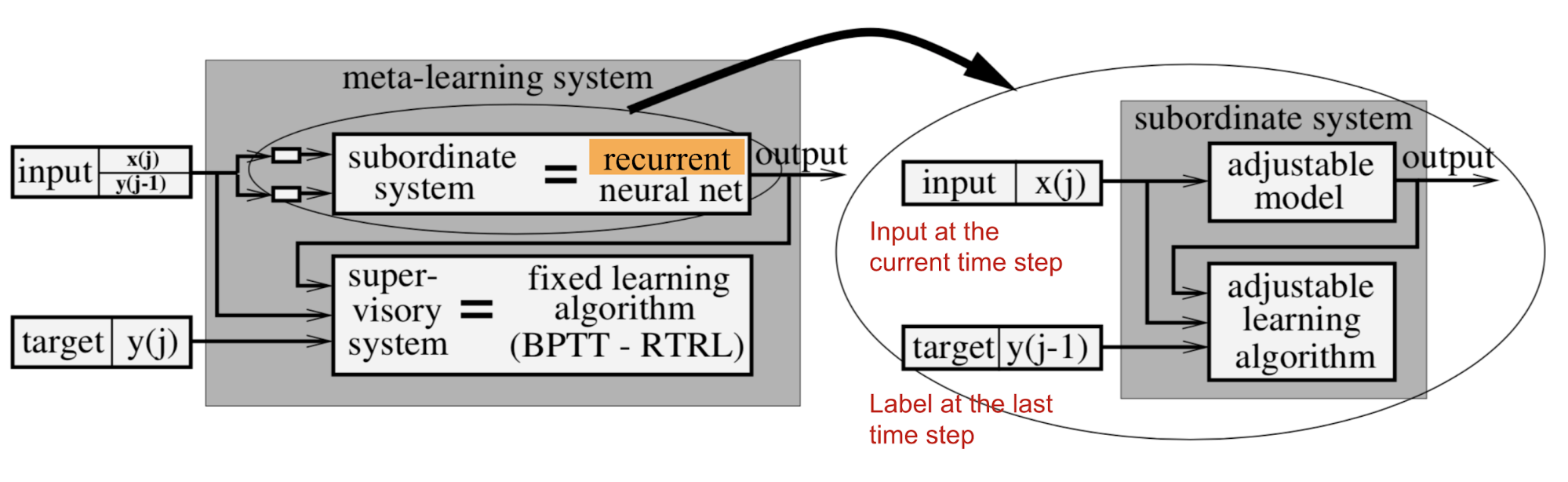Fig. 1. The meta-learning system consists of the supervisory and the subordinate systems. The subordinate system is a recurrent neural network that takes as input both the observation at the current time step, $$x_t$$ and the label at the last time step, $$y_{t-1}$$. (Image source: Hochreiter et al., 2001)

Hochreiter’s meta-learning model is a recurrent network with LSTM cell. LSTM is a good choice because it can internalize a history of inputs and tune its own weights effectively through BPTT. The training data contains $$K$$ sequences and each sequence is consist of $$N$$ samples generated by a target function $$f_k(.), k=1, \dots, K$$,

$\{\text{input: }(\mathbf{x}^k_i, \mathbf{y}^k_{i-1}) \to \text{label: }\mathbf{y}^k_i\}_{i=1}^N \text{ where }\mathbf{y}^k_i = f_k(\mathbf{x}^k_i)$

Noted that the last label $$\mathbf{y}^k_{i-1}$$ is also provided as an auxiliary input so that the function can learn the presented mapping.

In the experiment of decoding two-dimensional quadratic functions, $$a x_1^2 + b x_2^2 + c x_1 x_2 + d x_1 + e x_2 + f$$, with coefficients $$a$$-$$f$$ are randomly sampled from [-1, 1], this meta-learning system was able to approximate the function after seeing only ~35 examples.

Proposal in 2016

In the modern days of DL, Wang et al. (2016) and Duan et al. (2017) simultaneously proposed the very similar idea of Meta-RL (it is called RL^2 in the second paper). A meta-RL model is trained over a distribution of MDPs, and at test time, it is able to learn to solve a new task quickly. The goal of meta-RL is ambitious, taking one step further towards general algorithms.

Define Meta-RL

Meta Reinforcement Learning, in short, is to do meta-learning in the field of reinforcement learning. Usually the train and test tasks are different but drawn from the same family of problems; i.e., experiments in the papers included multi-armed bandit with different reward probabilities, mazes with different layouts, same robots but with different physical parameters in simulator, and many others.

Formulation

Let’s say we have a distribution of tasks, each formularized as an MDP (Markov Decision Process), $$M_i \in \mathcal{M}$$. An MDP is determined by a 4-tuple, $$M_i= \langle \mathcal{S}, \mathcal{A}, P_i, R_i \rangle$$:

Symbol Meaning
$$\mathcal{S}$$ A set of states.
$$\mathcal{A}$$ A set of actions.
$$P_i: \mathcal{S} \times \mathcal{A} \times \mathcal{S} \to \mathbb{R}_{+}$$ Transition probability function.
$$R_i: \mathcal{S} \times \mathcal{A} \to \mathbb{R}$$ Reward function.

(RL^2 paper adds an extra parameter, horizon $$T$$, into the MDP tuple to emphasize that each MDP should have a finite horizon.)

Note that common state $$\mathcal{S}$$ and action space $$\mathcal{A}$$ are used above, so that a (stochastic) policy: $$\pi_\theta: \mathcal{S} \times \mathcal{A} \to \mathbb{R}_{+}$$ would get inputs compatible across different tasks. The test tasks are sampled from the same distribution $$\mathcal{M}$$ or slightly modified version.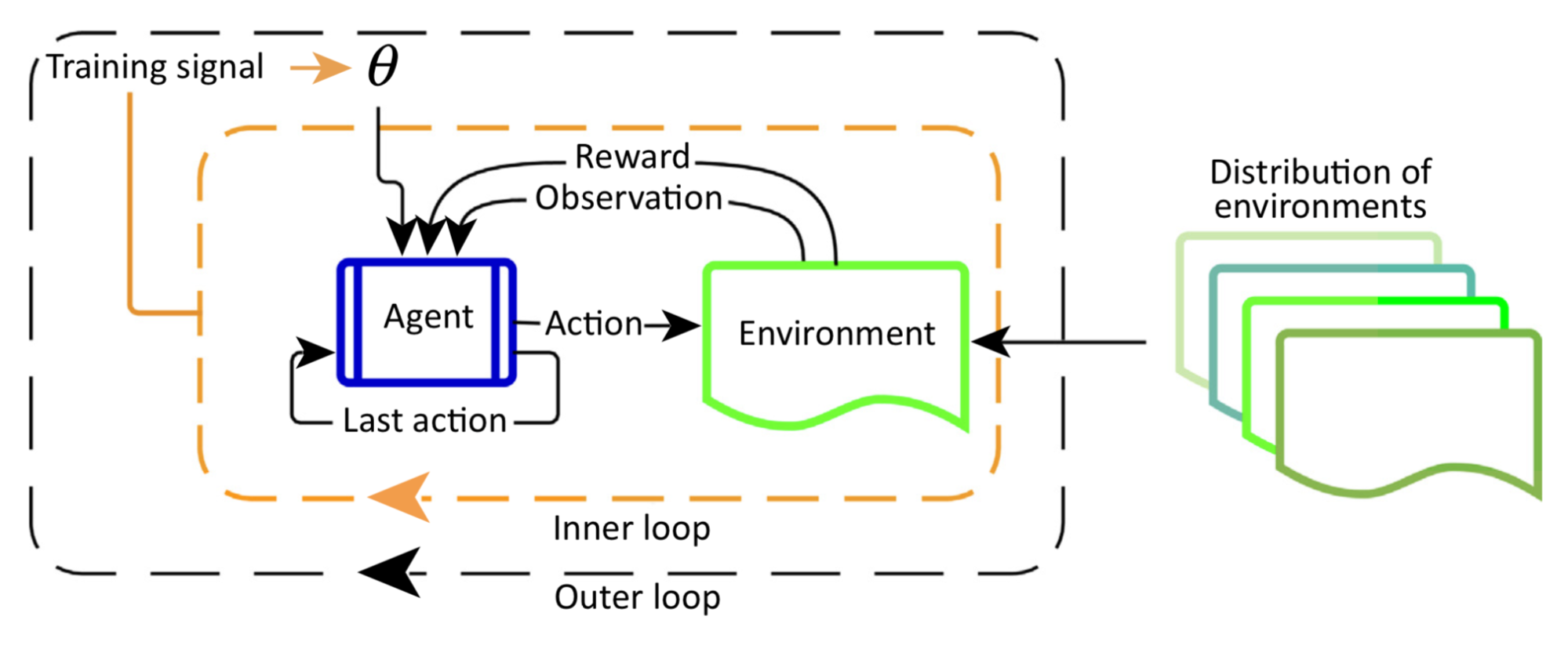Fig. 2. Illustration of meta-RL, containing two optimization loops. The outer loop samples a new environment in every iteration and adjusts parameters that determine the agent’s behavior. In the inner loop, the agent interacts with the environment and optimizes for the maximal reward. (Image source: Botvinick, et al. 2019

Main Differences from RL

The overall configure of meta-RL is very similar to an ordinary RL algorithm, except that the last reward $$r_{t-1}$$ and the last action $$a_{t-1}$$ are also incorporated into the policy observation in addition to the current state $$s_t$$.

• In RL: $$\pi_\theta(s_t) \to$$ a distribution over $$\mathcal{A}$$
• In meta-RL: $$\pi_\theta(a_{t-1}, r_{t-1}, s_t) \to$$ a distribution over $$\mathcal{A}$$

The intention of this design is to feed a history into the model so that the policy can internalize the dynamics between states, rewards, and actions in the current MDP and adjust its strategy accordingly. This is well aligned with the setup in Hochreiter’s system. Both meta-RL and RL^2 implemented an LSTM policy and the LSTM’s hidden states serve as a memory for tracking characteristics of the trajectories. Because the policy is recurrent, there is no need to feed the last state as inputs explicitly.

The training procedure works as follows:

1. Sample a new MDP, $$M_i \sim \mathcal{M}$$;
2. Reset the hidden state of the model;
3. Collect multiple trajectories and update the model weights;
4. Repeat from step 1.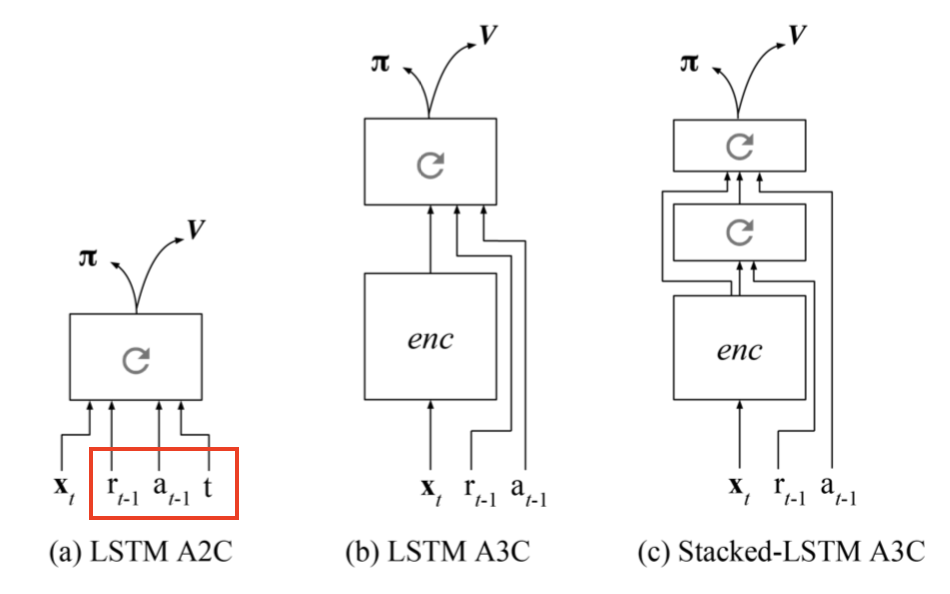Fig. 3. In the meta-RL paper, different actor-critic architectures all use a recurrent model. Last reward and last action are additional inputs. The observation is fed into the LSTM either as a one-hot vector or as an embedding vector after passed through an encoder model. (Image source: Wang et al., 2016)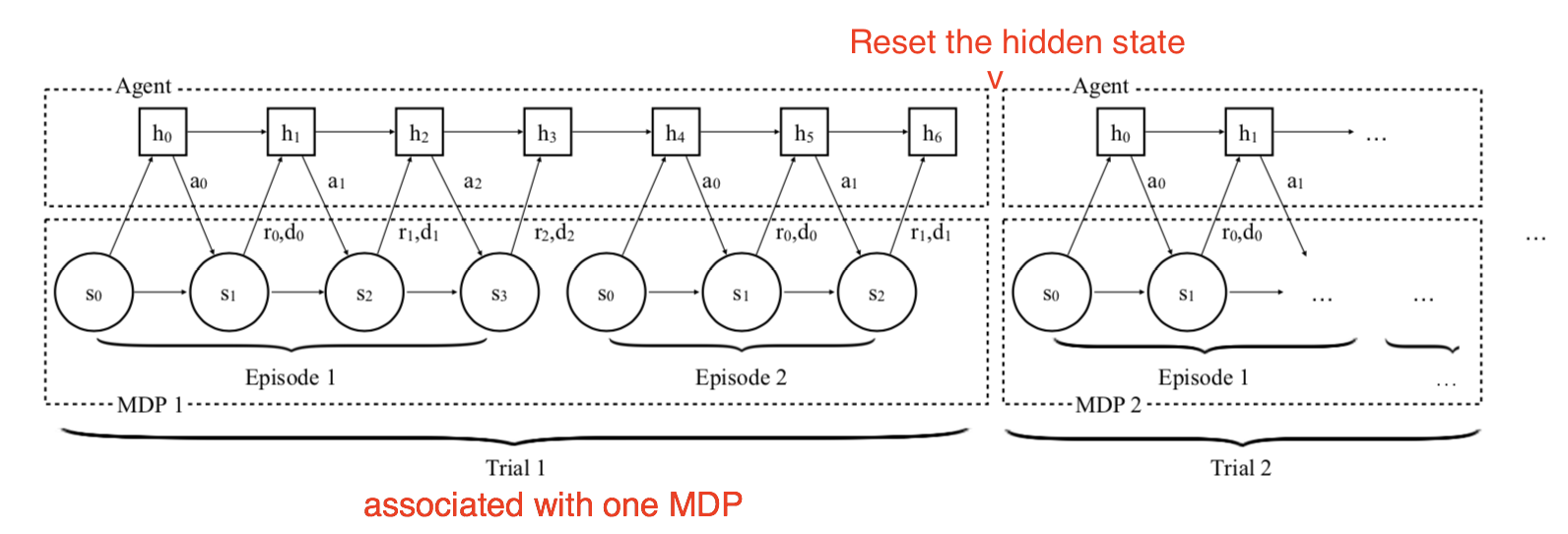Fig. 4. As described in the RL^2 paper, illustration of the procedure of the model interacting with a series of MDPs in training time . (Image source: Duan et al., 2017)

Key Components

There are three key components in Meta-RL:

A Model with Memory
A recurrent neural network maintains a hidden state. Thus, it could acquire and memorize the knowledge about the current task by updating the hidden state during rollouts. Without memory, meta-RL would not work.

Meta-learning Algorithm
A meta-learning algorithm refers to how we can update the model weights to optimize for the purpose of solving an unseen task fast at test time. In both Meta-RL and RL^2 papers, the meta-learning algorithm is the ordinary gradient descent update of LSTM with hidden state reset between a switch of MDPs.

A Distribution of MDPs
While the agent is exposed to a variety of environments and tasks during training, it has to learn how to adapt to different MDPs.

According to Botvinick et al. (2019), one source of slowness in RL training is weak inductive bias ( = “a set of assumptions that the learner uses to predict outputs given inputs that it has not encountered”). As a general ML rule, a learning algorithm with weak inductive bias will be able to master a wider range of variance, but usually, will be less sample-efficient. Therefore, to narrow down the hypotheses with stronger inductive biases help improve the learning speed.

In meta-RL, we impose certain types of inductive biases from the task distribution and store them in memory. Which inductive bias to adopt at test time depends on the algorithm. Together, these three key components depict a compelling view of meta-RL: Adjusting the weights of a recurrent network is slow but it allows the model to work out a new task fast with its own RL algorithm implemented in its internal activity dynamics.

Meta-RL interestingly and not very surprisingly matches the ideas in the AI-GAs (“AI-Generating Algorithms”) paper by Jeff Clune (2019). He proposed that one efficient way towards building general AI is to make learning as automatic as possible. The AI-GAs approach involves three pillars: (1) meta-learning architectures, (2) meta-learning algorithms, and (3) automatically generated environments for effective learning.

The topic of designing good recurrent network architectures is a bit too broad to be discussed here, so I will skip it. Next, let’s look further into another two components: meta-learning algorithms in the context of meta-RL and how to acquire a variety of training MDPs.

Meta-Learning Algorithms for Meta-RL

My previous post on meta-learning has covered several classic meta-learning algorithms. Here I’m gonna include more related to RL.

Optimizing Model Weights for Meta-learning

Both MAML (Finn, et al. 2017) and Reptile (Nichol et al., 2018) are methods on updating model parameters in order to achieve good generalization performance on new tasks. See an earlier post section on MAML and Reptile.

Meta-learning Hyperparameters

The return function in an RL problem, $$G_t^{(n)}$$ or $$G_t^\lambda$$, involves a few hyperparameters that are often set heuristically, like the discount factor $$\gamma$$ and the bootstrapping parameter $$\lambda$$. Meta-gradient RL (Xu et al., 2018) considers them as meta-parameters, $$\eta=\{\gamma, \lambda \}$$, that can be tuned and learned online while an agent is interacting with the environment. Therefore, the return becomes a function of $$\eta$$ and dynamically adapts itself to a specific task over time.

\begin{aligned} G_\eta^{(n)}(\tau_t) &= R_{t+1} + \gamma R_{t+2} + \dots + \gamma^{n-1}R_{t+n} + \gamma^n v_\theta(s_{t+n}) & \scriptstyle{\text{; n-step return}} \\ G_\eta^{\lambda}(\tau_t) &= (1-\lambda) \sum_{n=1}^\infty \lambda^{n-1} G_\eta^{(n)} & \scriptstyle{\text{; λ-return, mixture of n-step returns}} \end{aligned}

During training, we would like to update the policy parameters with gradients as a function of all the information in hand, $$\theta' = \theta + f(\tau, \theta, \eta)$$, where $$\theta$$ are the current model weights, $$\tau$$ is a sequence of trajectories, and $$\eta$$ are the meta-parameters.

Meanwhile, let’s say we have a meta-objective function $$J(\tau, \theta, \eta)$$ as a performance measure. The training process follows the principle of online cross-validation, using a sequence of consecutive experiences:

1. Starting with parameter $$\theta$$, the policy $$\pi_\theta$$ is updated on the first batch of samples $$\tau$$, resulting in $$\theta'$$.
2. Then we continue running the policy $$\pi_{\theta'}$$ to collect a new set of experiences $$\tau'$$, just following $$\tau$$ consecutively in time. The performance is measured as $$J(\tau', \theta', \bar{\eta})$$ with a fixed meta-parameter $$\bar{\eta}$$.
3. The gradient of meta-objective $$J(\tau', \theta', \bar{\eta})$$ w.r.t. $$\eta$$ is used to update $$\eta$$:
\begin{aligned} \Delta \eta &= -\beta \frac{\partial J(\tau', \theta', \bar{\eta})}{\partial \eta} \\ &= -\beta \frac{\partial J(\tau', \theta', \bar{\eta})}{\partial \theta'} \frac{d\theta'}{d\eta} & \scriptstyle{\text{ ; single variable chain rule.}} \\ &= -\beta \frac{\partial J(\tau', \theta', \bar{\eta})}{\partial \theta'} \frac{\partial (\theta + f(\tau, \theta, \eta))}{\partial\eta} \\ &= -\beta \frac{\partial J(\tau', \theta', \bar{\eta})}{\partial \theta'} \Big(\frac{d\theta}{d\eta} + \frac{\partial f(\tau, \theta, \eta)}{\partial\theta}\frac{d\theta}{d\eta} + \frac{\partial f(\tau, \theta, \eta)}{\partial\eta}\frac{d\eta}{d\eta} \Big) & \scriptstyle{\text{; multivariable chain rule.}}\\ &= -\beta \frac{\partial J(\tau', \theta', \bar{\eta})}{\partial \theta'} \Big( \color{red}{\big(\mathbf{I} + \frac{\partial f(\tau, \theta, \eta)}{\partial\theta}\big)}\frac{d\theta}{d\eta} + \frac{\partial f(\tau, \theta, \eta)}{\partial\eta}\Big) & \scriptstyle{\text{; secondary gradient term in red.}} \end{aligned}

where $$\beta$$ is the learning rate for $$\eta$$.

The meta-gradient RL algorithm simplifies the computation by setting the secondary gradient term to zero, $$\mathbf{I} + \partial g(\tau, \theta, \eta)/\partial\theta = 0$$ — this choice prefers the immediate effect of the meta-parameters $$\eta$$ on the parameters $$\theta$$. Eventually we get:

$\Delta \eta = -\beta \frac{\partial J(\tau', \theta', \bar{\eta})}{\partial \theta'} \frac{\partial f(\tau, \theta, \eta)}{\partial\eta}$

Experiments in the paper adopted the meta-objective function same as $$TD(\lambda)$$ algorithm, minimizing the error between the approximated value function $$v_\theta(s)$$ and the $$\lambda$$-return:

\begin{aligned} J(\tau, \theta, \eta) &= (G^\lambda_\eta(\tau) - v_\theta(s))^2 \\ J(\tau', \theta', \bar{\eta}) &= (G^\lambda_{\bar{\eta}}(\tau') - v_{\theta'}(s'))^2 \end{aligned}

Meta-learning the Loss Function

In policy gradient algorithms, the expected total reward is maximized by updating the policy parameters $$\theta$$ in the direction of estimated gradient (Schulman et al., 2016),

$g = \mathbb{E}[\sum_{t=0}^\infty \Psi_t \nabla_\theta \log \pi_\theta (a_t \mid s_t)]$

where the candidates for $$\Psi_t$$ include the trajectory return $$G_t$$, the Q value $$Q(s_t, a_t)$$, or the advantage value $$A(s_t, a_t)$$. The corresponding surrogate loss function for the policy gradient can be reverse-engineered:

$L_\text{pg} = \mathbb{E}[\sum_{t=0}^\infty \Psi_t \log \pi_\theta (a_t \mid s_t)]$

This loss function is a measure over a history of trajectories, $$(s_0, a_0, r_0, \dots, s_t, a_t, r_t, \dots)$$. Evolved Policy Gradient (EPG; Houthooft, et al, 2018) takes a step further by defining the policy gradient loss function as a temporal convolution (1-D convolution) over the agent’s past experience, $$L_\phi$$. The parameters $$\phi$$ of the loss function network are evolved in a way that an agent can achieve higher returns.

Similar to many meta-learning algorithms, EPG has two optimization loops:

• In the internal loop, an agent learns to improve its policy $$\pi_\theta$$.
• In the outer loop, the model updates the parameters $$\phi$$ of the loss function $$L_\phi$$. Because there is no explicit way to write down a differentiable equation between the return and the loss, EPG turned to Evolutionary Strategies (ES).

A general idea is to train a population of $$N$$ agents, each of them is trained with the loss function $$L_{\phi + \sigma \epsilon_i}$$ parameterized with $$\phi$$ added with a small Gaussian noise $$\epsilon_i \sim \mathcal{N}(0, \mathbf{I})$$ of standard deviation $$\sigma$$. During the inner loop’s training, EPG tracks a history of experience and updates the policy parameters according to the loss function $$L_{\phi + \sigma\epsilon_i}$$ for each agent:

$\theta_i \leftarrow \theta - \alpha_\text{in} \nabla_\theta L_{\phi + \sigma \epsilon_i} (\pi_\theta, \tau_{t-K, \dots, t})$

where $$\alpha_\text{in}$$ is the learning rate of the inner loop and $$\tau_{t-K, \dots, t}$$ is a sequence of $$M$$ transitions up to the current time step $$t$$.

Once the inner loop policy is mature enough, the policy is evaluated by the mean return $$\bar{G}_{\phi+\sigma\epsilon_i}$$ over multiple randomly sampled trajectories. Eventually, we are able to estimate the gradient of $$\phi$$ according to NES numerically (Salimans et al, 2017). While repeating this process, both the policy parameters $$\theta$$ and the loss function weights $$\phi$$ are being updated simultaneously to achieve higher returns.

$\phi \leftarrow \phi + \alpha_\text{out} \frac{1}{\sigma N} \sum_{i=1}^N \epsilon_i G_{\phi+\sigma\epsilon_i}$

where $$\alpha_\text{out}$$ is the learning rate of the outer loop.

In practice, the loss $$L_\phi$$ is bootstrapped with an ordinary policy gradient (such as REINFORCE or PPO) surrogate loss $$L_\text{pg}$$, $$\hat{L} = (1-\alpha) L_\phi + \alpha L_\text{pg}$$. The weight $$\alpha$$ is annealing from 1 to 0 gradually during training. At test time, the loss function parameter $$\phi$$ stays fixed and the loss value is computed over a history of experience to update the policy parameters $$\theta$$.

Meta-learning the Exploration Strategies

The exploitation vs exploration dilemma is a critical problem in RL. Common ways to do exploration include $$\epsilon$$-greedy, random noise on actions, or stochastic policy with built-in randomness on the action space.

MAESN (Gupta et al, 2018) is an algorithm to learn structured action noise from prior experience for better and more effective exploration. Simply adding random noise on actions cannot capture task-dependent or time-correlated exploration strategies. MAESN changes the policy to condition on a per-task random variable $$z_i \sim \mathcal{N}(\mu_i, \sigma_i)$$, for $$i$$-th task $$M_i$$, so we would have a policy $$a \sim \pi_\theta(a\mid s, z_i)$$. The latent variable $$z_i$$ is sampled once and fixed during one episode. Intuitively, the latent variable determines one type of behavior (or skills) that should be explored more at the beginning of a rollout and the agent would adjust its actions accordingly. Both the policy parameters and latent space are optimized to maximize the total task rewards. In the meantime, the policy learns to make use of the latent variables for exploration.

In addition, the loss function includes a KL divergence between the learned latent variable and a unit Gaussian prior, $$D_\text{KL}(\mathcal{N}(\mu_i, \sigma_i)\|\mathcal{N}(0, \mathbf{I}))$$. On one hand, it restricts the learned latent space not too far from a common prior. On the other hand, it creates the variational evidence lower bound (ELBO) for the reward function. Interestingly the paper found that $$(\mu_i, \sigma_i)$$ for each task are usually close to the prior at convergence.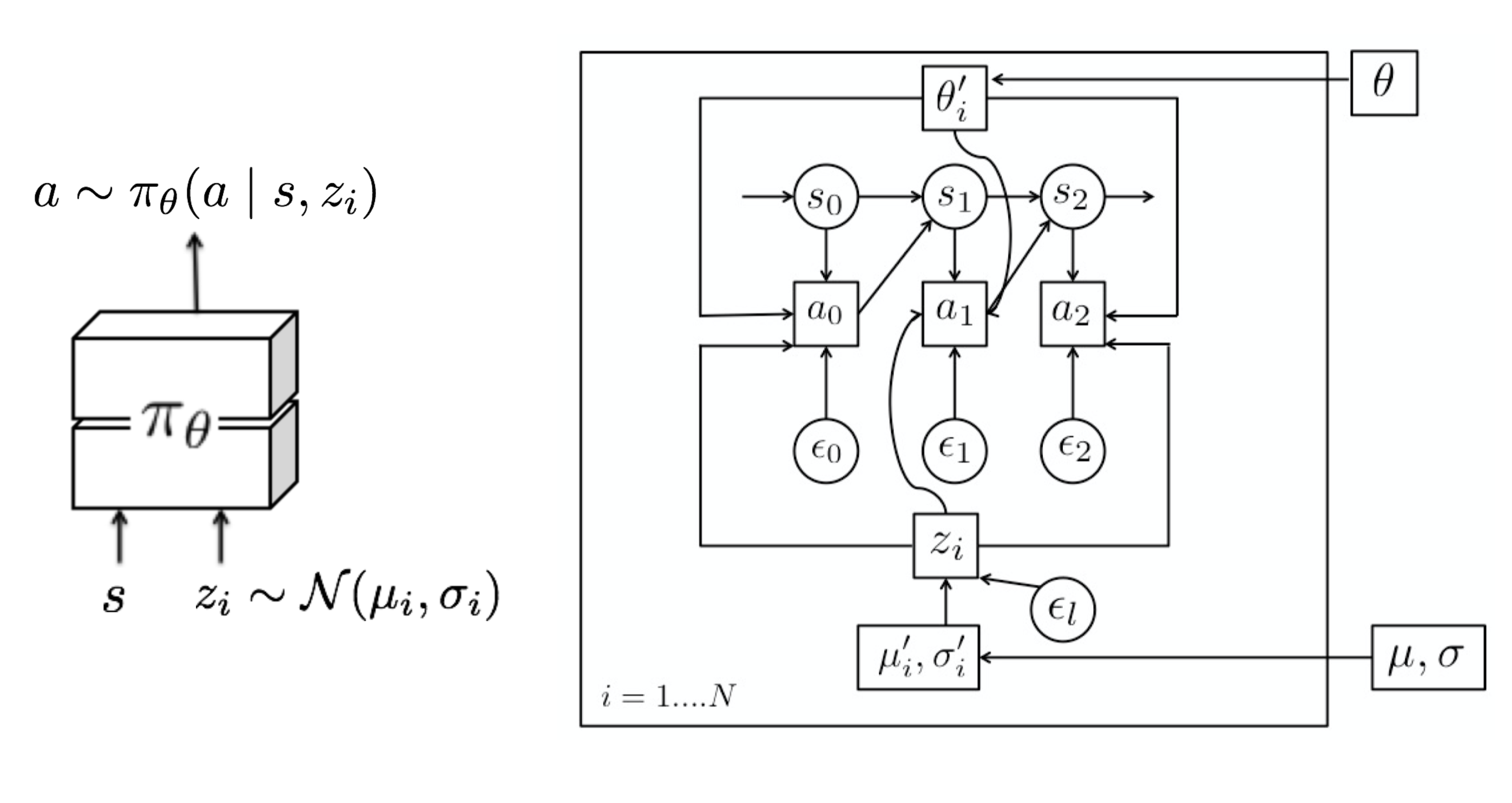Fig. 5. The policy is conditioned on a latent variable variable $$z_i \sim \mathcal{N}(\mu, \sigma)$$ that is sampled once every episode. Each task has different hyperparameters for the latent variable distribution, $$(\mu_i, \sigma_i)$$ and they are optimized in the outer loop. (Image source: Gupta et al, 2018)

Episodic Control

A major criticism of RL is on its sample inefficiency. A large number of samples and small learning steps are required for incremental parameter adjustment in RL in order to maximize generalization and avoid catastrophic forgetting of earlier learning (Botvinick et al., 2019).

Episodic control (Lengyel & Dayan, 2008) is proposed as a solution to avoid forgetting and improve generalization while training at a faster speed. It is partially inspired by hypotheses on instance-based hippocampal learning.

An episodic memory keeps explicit records of past events and uses these records directly as point of reference for making new decisions (i.e. just like metric-based meta-learning). In MFEC (Model-Free Episodic Control; Blundell et al., 2016), the memory is modeled as a big table, storing the state-action pair $$(s, a)$$ as key and the corresponding Q-value $$Q_\text{EC}(s, a)$$ as value. When receiving a new observation $$s$$, the Q value is estimated in an non-parametric way as the average Q-value of top $$k$$ most similar samples:

$\hat{Q}_\text{EC}(s, a) = \begin{cases} Q_\text{EC}(s, a) & \text{if } (s,a) \in Q_\text{EC}, \\ \frac{1}{k} \sum_{i=1}^k Q(s^{(i)}, a) & \text{otherwise} \end{cases}$

where $$s^{(i)}, i=1, \dots, k$$ are top $$k$$ states with smallest distances to the state $$s$$. Then the action that yields the highest estimated Q value is selected. Then the memory table is updated according to the return received at $$s_t$$:

$Q_\text{EC}(s, a) \leftarrow \begin{cases} \max\{Q_\text{EC}(s_t, a_t), G_t\} & \text{if } (s,a) \in Q_\text{EC}, \\ G_t & \text{otherwise} \end{cases}$

As a tabular RL method, MFEC suffers from large memory consumption and a lack of ways to generalize among similar states. The first one can be fixed with an LRU cache. Inspired by metric-based meta-learning, especially Matching Networks (Vinyals et al., 2016), the generalization problem is improved in a follow-up algorithm, NEC (Neural Episodic Control; Pritzel et al., 2016).

The episodic memory in NEC is a Differentiable Neural Dictionary (DND), where the key is a convolutional embedding vector of input image pixels and the value stores estimated Q value. Given an inquiry key, the output is a weighted sum of values of top similar keys, where the weight is a normalized kernel measure between the query key and the selected key in the dictionary. This sounds like a hard attention machanism.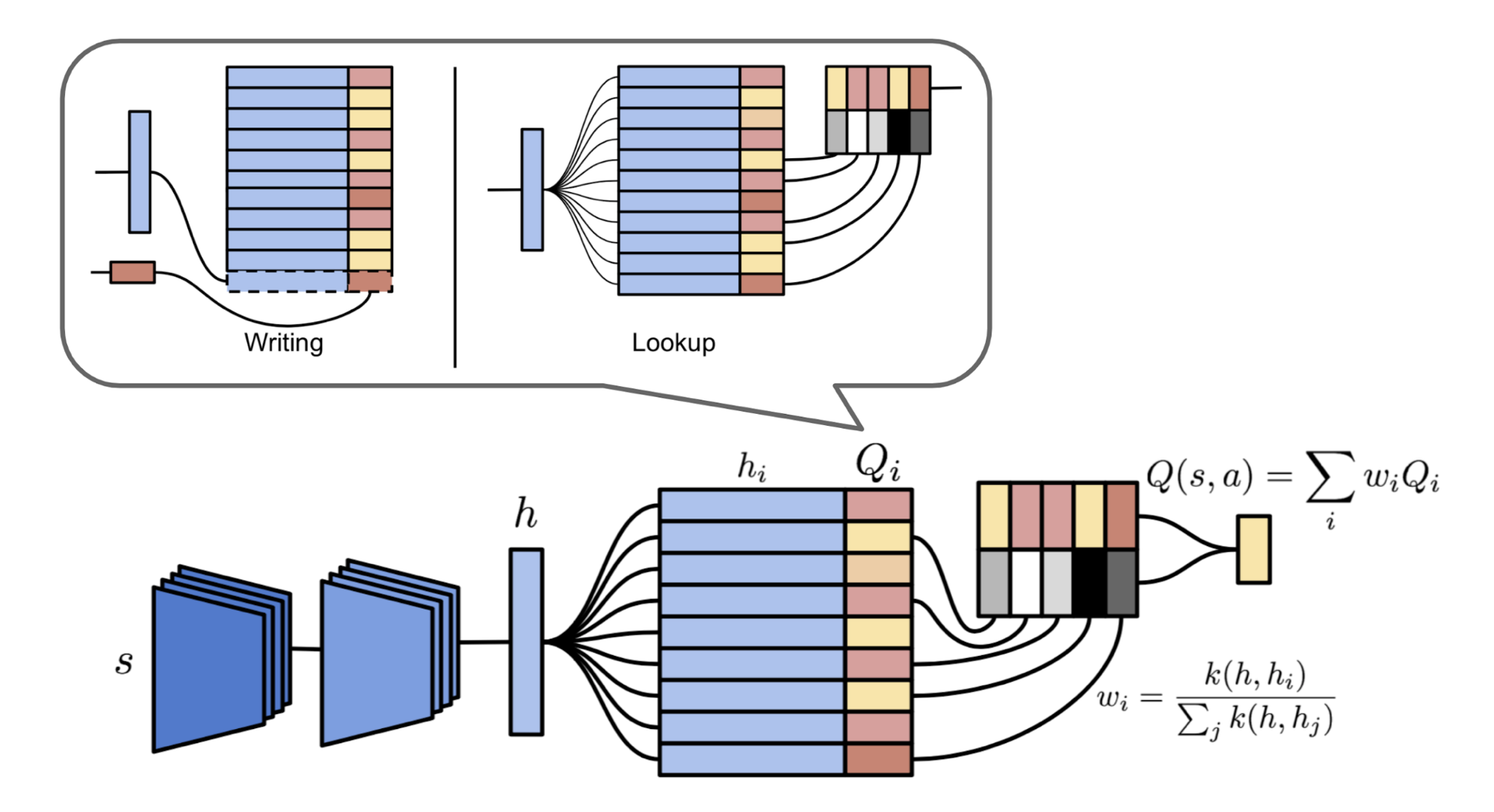Fig. 6 Illustrations of episodic memory module in NEC and two operations on a differentiable neural dictionary. (Image source: Pritzel et al., 2016)

Further, Episodic LSTM (Ritter et al., 2018) enhances the basic LSTM architecture with a DND episodic memory, which stores task context embeddings as keys and the LSTM cell states as values. The stored hidden states are retrieved and added directly to the current cell state through the same gating mechanism within LSTM: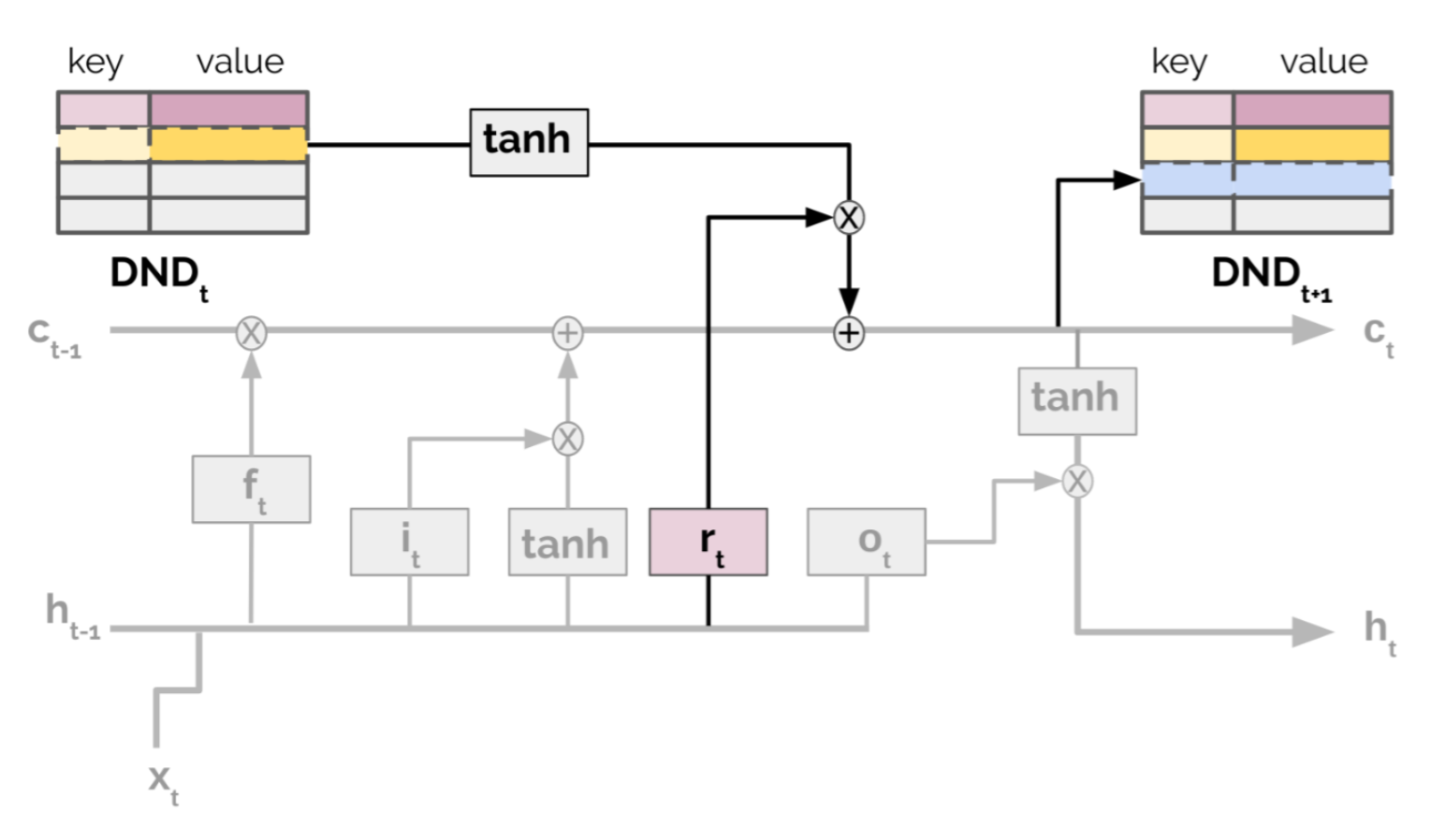Fig. 7. Illustration of the episodic LSTM architecture. The additional structure of episodic memory is in bold. (Image source: Ritter et al., 2018)

\begin{aligned} \mathbf{c}_t &= \mathbf{i}_t \circ \mathbf{c}_\text{in} + \mathbf{f}_t \circ \mathbf{c}_{t-1} + \color{green}{\mathbf{r}_t \circ \mathbf{c}_\text{ep}} &\\ \mathbf{i}_t &= \sigma(\mathbf{W}_{i} \cdot [\mathbf{h}_{t-1}, \mathbf{x}_t] + \mathbf{b}_i) & \scriptstyle{\text{; input gate}} \\ \mathbf{f}_t &= \sigma(\mathbf{W}_{f} \cdot [\mathbf{h}_{t-1}, \mathbf{x}_t] + \mathbf{b}_f) & \scriptstyle{\text{; forget gate}} \\ \color{green}{\mathbf{r}_t} & \color{green}{=} \color{green}{\sigma(\mathbf{W}_{r} \cdot [\mathbf{h}_{t-1}, \mathbf{x}_t] + \mathbf{b}_r)} & \scriptstyle{\text{; reinstatement gate}} \end{aligned}

where $$\mathbf{c}_t$$ and $$\mathbf{h}_t$$ are hidden and cell state at time $$t$$; $$\mathbf{i}_t$$, $$\mathbf{f}_t$$ and $$\mathbf{r}_t$$ are input, forget and reinstatement gates, respectively; $$\mathbf{c}_\text{ep}$$ is the retrieved cell state from episodic memory. The newly added episodic memory components are marked in green.

This architecture provides a shortcut to the prior experience through context-based retrieval. Meanwhile, explicitly saving the task-dependent experience in an external memory avoids forgetting. In the paper, all the experiments have manually designed context vectors. How to construct an effective and efficient format of task context embeddings for more free-formed tasks would be an interesting topic.

Overall the capacity of episodic control is limited by the complexity of the environment. It is very rare for an agent to repeatedly visit exactly the same states in a real-world task, so properly encoding the states is critical. The learned embedding space compresses the observation data into a lower dimension space and, in the meantime, two states being close in this space are expected to demand similar strategies.

Among three key components, how to design a proper distribution of tasks is the less studied and probably the most specific one to meta-RL itself. As described above, each task is a MDP: $$M_i = \langle \mathcal{S}, \mathcal{A}, P_i, R_i \rangle \in \mathcal{M}$$. We can build a distribution of MDPs by modifying:

• The reward configuration: Among different tasks, same behavior might get rewarded differently according to $$R_i$$.
• Or, the environment: The transition function $$P_i$$ can be reshaped by initializing the environment with varying shifts between states.

Randomizing parameters in a simulator is an easy way to obtain tasks with modified transition functions. If interested in learning further, check my last post on domain randomization.

Evolutionary Algorithm on Environment Generation

Evolutionary algorithm is a gradient-free heuristic-based optimization method, inspired by natural selection. A population of solutions follows a loop of evaluation, selection, reproduction, and mutation. Eventually, good solutions survive and thus get selected.

POET (Wang et al, 2019), a framework based on the evolutionary algorithm, attempts to generate tasks while the problems themselves are being solved. The implementation of POET is only specifically designed for a simple 2D bipedal walker environment but points out an interesting direction. It is noteworthy that the evolutionary algorithm has had some compelling applications in Deep Learning like EPG and PBT (Population-Based Training; Jaderberg et al, 2017).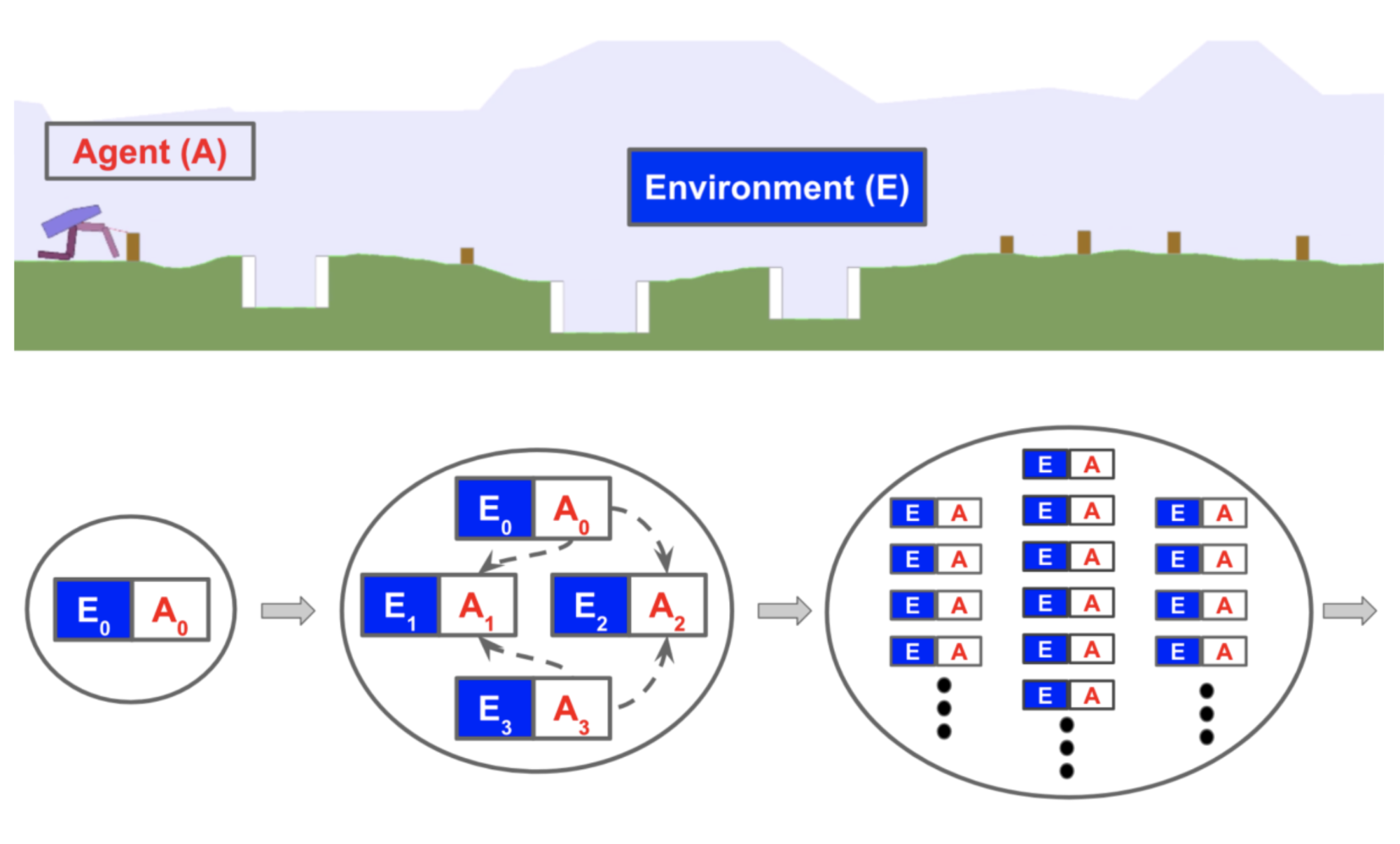Fig. 8. An example bipedal walking environment (top) and an overview of POET (bottom). (Image source: POET blog post)

The 2D bipedal walking environment is evolving: from a simple flat surface to a much more difficult trail with potential gaps, stumps, and rough terrains. POET pairs the generation of environmental challenges and the optimization of agents together so as to (a) select agents that can resolve current challenges and (b) evolve environments to be solvable. The algorithm maintains a list of environment-agent pairs and repeats the following:

1. Mutation: Generate new environments from currently active environments. Note that here types of mutation operations are created just for bipedal walker and a new environment would demand a new set of configurations.
2. Optimization: Train paired agents within their respective environments.
3. Selection: Periodically attempt to transfer current agents from one environment to another. Copy and update the best performing agent for every environment. The intuition is that skills learned in one environment might be helpful for a different environment.

The procedure above is quite similar to PBT, but PBT mutates and evolves hyperparameters instead. To some extent, POET is doing domain randomization, as all the gaps, stumps and terrain roughness are controlled by some randomization probability parameters. Different from DR, the agents are not exposed to a fully randomized difficult environment all at once, but instead they are learning gradually with a curriculum configured by the evolutionary algorithm.

Learning with Random Rewards

An MDP without a reward function $$R$$ is known as a Controlled Markov process (CMP). Given a predefined CMP, $$\langle \mathcal{S}, \mathcal{A}, P\rangle$$, we can acquire a variety of tasks by generating a collection of reward functions $$\mathcal{R}$$ that encourage the training of an effective meta-learning policy.

Gupta et al. (2018) proposed two unsupervised approaches for growing the task distribution in the context of CMP. Assuming there is an underlying latent variable $$z \sim p(z)$$ associated with every task, it parameterizes/determines a reward function: $$r_z(s) = \log D(z|s)$$, where a “discriminator” function $$D(.)$$ is used to extract the latent variable from the state. The paper described two ways to construct a discriminator function:

• Sample random weights $$\phi_\text{rand}$$ of the discriminator, $$D_{\phi_\text{rand}}(z \mid s)$$.
• Learn a discriminator function to encourage diversity-driven exploration. This method is introduced in more details in another sister paper “DIAYN” (Eysenbach et al., 2018).

DIAYN, short for “Diversity is all you need”, is a framework to encourage a policy to learn useful skills without a reward function. It explicitly models the latent variable $$z$$ as a skill embedding and makes the policy conditioned on $$z$$ in addition to state $$s$$, $$\pi_\theta(a \mid s, z)$$. (Ok, this part is same as MAESN unsurprisingly, as the papers are from the same group.) The design of DIAYN is motivated by a few hypotheses:

• Skills should be diverse and lead to visitations of different states. → maximize the mutual information between states and skills, $$I(S; Z)$$
• Skills should be distinguishable by states, not actions. → minimize the mutual information between actions and skills, conditioned on states $$I(A; Z \mid S)$$

The objective function to maximize is as follows, where the policy entropy is also added to encourage diversity:

\begin{aligned} \mathcal{F}(\theta) &= I(S; Z) + H[A \mid S] - I(A; Z \mid S) & \\ &= (H(Z) - H(Z \mid S)) + H[A \mid S] - (H[A\mid S] - H[A\mid S, Z]) & \\ &= H[A\mid S, Z] \color{green}{- H(Z \mid S) + H(Z)} & \\ &= H[A\mid S, Z] + \mathbb{E}_{z\sim p(z), s\sim\rho(s)}[\log p(z \mid s)] - \mathbb{E}_{z\sim p(z)}[\log p(z)] & \scriptstyle{\text{; can infer skills from states & p(z) is diverse.}} \\ &\ge H[A\mid S, Z] + \mathbb{E}_{z\sim p(z), s\sim\rho(s)}[\color{red}{\log D_\phi(z \mid s) - \log p(z)}] & \scriptstyle{\text{; according to Jensen's inequality; "pseudo-reward" in red.}} \end{aligned}

where $$I(.)$$ is mutual information and $$H[.]$$ is entropy measure. We cannot integrate all states to compute $$p(z \mid s)$$, so approximate it with $$D_\phi(z \mid s)$$ — that is the diversity-driven discriminator function.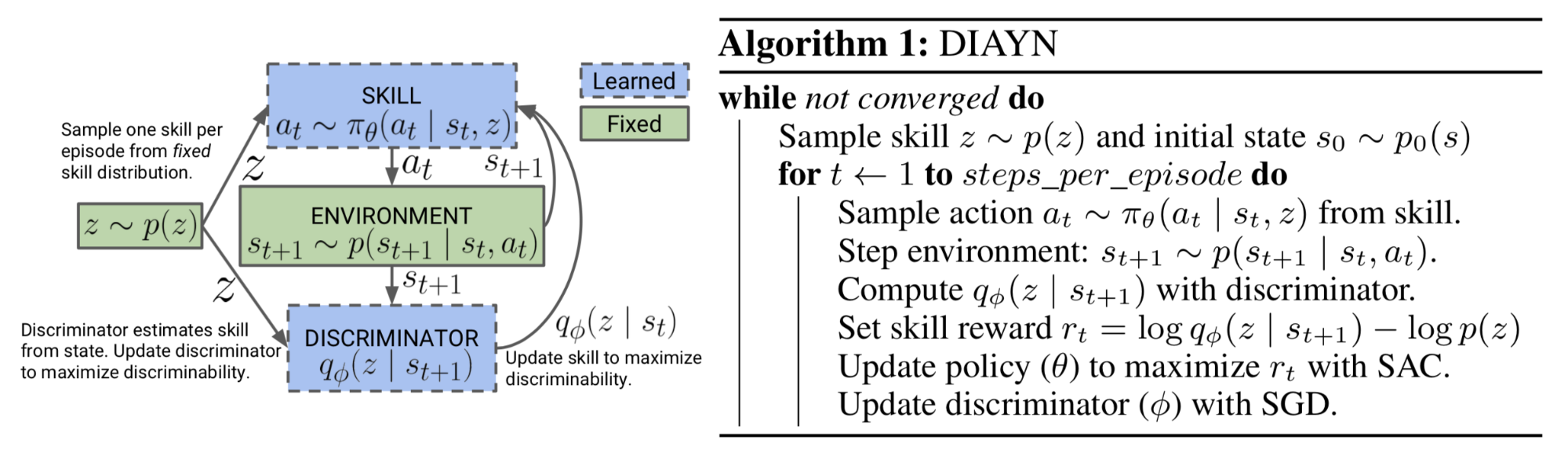Fig. 9. DIAYN Algorithm. (Image source: Eysenbach et al., 2019)

Once the discriminator function is learned, sampling a new MDP for training is strainght-forward: First, sample a latent variable, $$z \sim p(z)$$ and construct a reward function $$r_z(s) = \log(D(z \vert s))$$. Pairing the reward function with a predefined CMP creates a new MDP.

Cited as:

@article{weng2019metaRL,
title   = "Meta Reinforcement Learning",
author  = "Weng, Lilian",
journal = "lilianweng.github.io/lil-log",
year    = "2019",
url     = "http://lilianweng.github.io/lil-log/2019/06/23/meta-reinforcement-learning.html"
}

 Richard S. Sutton. “The Bitter Lesson.” March 13, 2019.

 Sepp Hochreiter, A. Steven Younger, and Peter R. Conwell. “Learning to learn using gradient descent.” Intl. Conf. on Artificial Neural Networks. 2001.

 Jane X Wang, et al. “Learning to reinforcement learn.” arXiv preprint arXiv:1611.05763 (2016).

 Yan Duan, et al. “RL $^ 2$: Fast Reinforcement Learning via Slow Reinforcement Learning.” ICLR 2017.

 Matthew Botvinick, et al. “Reinforcement Learning, Fast and Slow” Cell Review, Volume 23, Issue 5, P408-422, May 01, 2019.

 Jeff Clune. “AI-GAs: AI-generating algorithms, an alternate paradigm for producing general artificial intelligence” arXiv preprint arXiv:1905.10985 (2019).

 Zhongwen Xu, et al. “Meta-Gradient Reinforcement Learning” NIPS 2018.

 Rein Houthooft, et al. “Evolved Policy Gradients.” NIPS 2018.

 Tim Salimans, et al. “Evolution strategies as a scalable alternative to reinforcement learning.” arXiv preprint arXiv:1703.03864 (2017).

 Abhishek Gupta, et al. “Meta-Reinforcement Learning of Structured Exploration Strategies.” NIPS 2018.

 Alexander Pritzel, et al. “Neural episodic control.” Proc. Intl. Conf. on Machine Learning, Volume 70, 2017.

 Charles Blundell, et al. “Model-free episodic control.” arXiv preprint arXiv:1606.04460 (2016).

 Samuel Ritter, et al. “Been there, done that: Meta-learning with episodic recall.” ICML, 2018.

 Rui Wang et al. “Paired Open-Ended Trailblazer (POET): Endlessly Generating Increasingly Complex and Diverse Learning Environments and Their Solutions” arXiv preprint arXiv:1901.01753 (2019).

 Uber Engineering Blog: “POET: Endlessly Generating Increasingly Complex and Diverse Learning Environments and their Solutions through the Paired Open-Ended Trailblazer.” Jan 8, 2019.

 Abhishek Gupta, et al.“Unsupervised meta-learning for Reinforcement Learning” arXiv preprint arXiv:1806.04640 (2018).

 Eysenbach, Benjamin, et al. “Diversity is all you need: Learning skills without a reward function.” ICLR 2019.

 Max Jaderberg, et al. “Population Based Training of Neural Networks.” arXiv preprint arXiv:1711.09846 (2017).